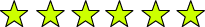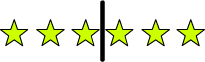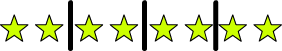# Fractions of a Number

Print Rate 0 stars Common Core
Lesson size:
Message preview:
Someone you know has shared lesson with you:

To play this lesson, click on the link below:

https://www.turtlediary.com/lesson/fractions-of-a-number.html

Hope you have a good experience with this site and recommend to your friends too.

Login to rate activities and track progress.
Login to rate activities and track progress.
What is
1
2
of 6?

To answer this question, let's first draw 6 stars.Since our denominator is 2, we need to split up these stars into 2 even groups:And since our numerator is 1, we need to look at one of the groups.So, 3 is
1
2
of 6 .

Let's take a look at a few examples of fractions of numbers:

## Example 1

What is
1
3
of 12?

Let's draw 12 stars and split them into 3 groups (because 3 is the denominator).Since the numerator is 1, we'll circle 1 group and count up those stars:There are 4 stars in 1 group.

So,
1
3
of 12 is 4.

## Example 2

What is
3
4
of 8?

We need to draw 8 stars and split them up into 4 groups (because 4 is the denominator).Since the numerator is 3, we'll circle 3 groups and count up those stars:6 stars are circled, so our answer is 6.

3
4
of 8 is 6.

## Example 3

What is
2
5
of 10?

Start with 10 stars and divide them into 5 groups (because the denominator is 5).

Then, circle 2 of these groups (because the numerator is 2).So,
2
5
of 10 is equal to 4.

## Fractions of a Number

• Fractions show pieces of a whole and consists of a numerator and a denominator.
• The denominator (the bottom number) tells how many total pieces there are and the numerator (the top number) tells how many of those pieces we are talking about.

## Similar Lessons

Become premium member to get unlimited access.